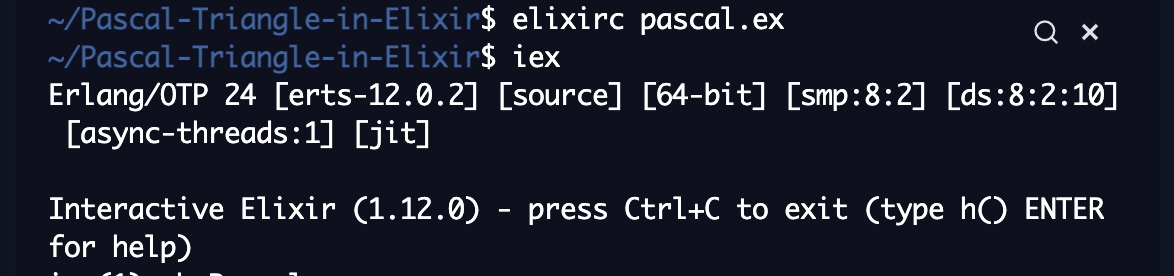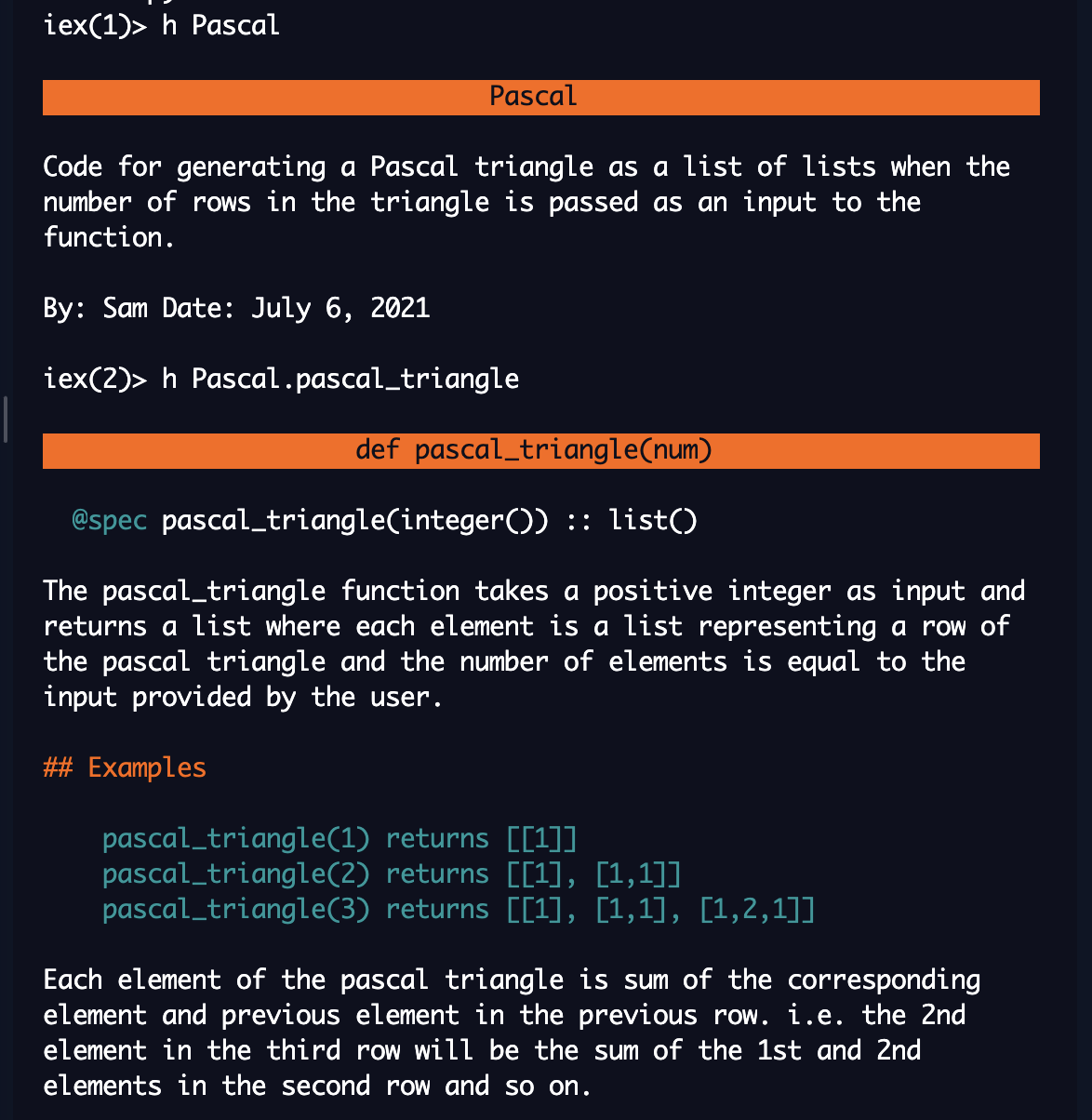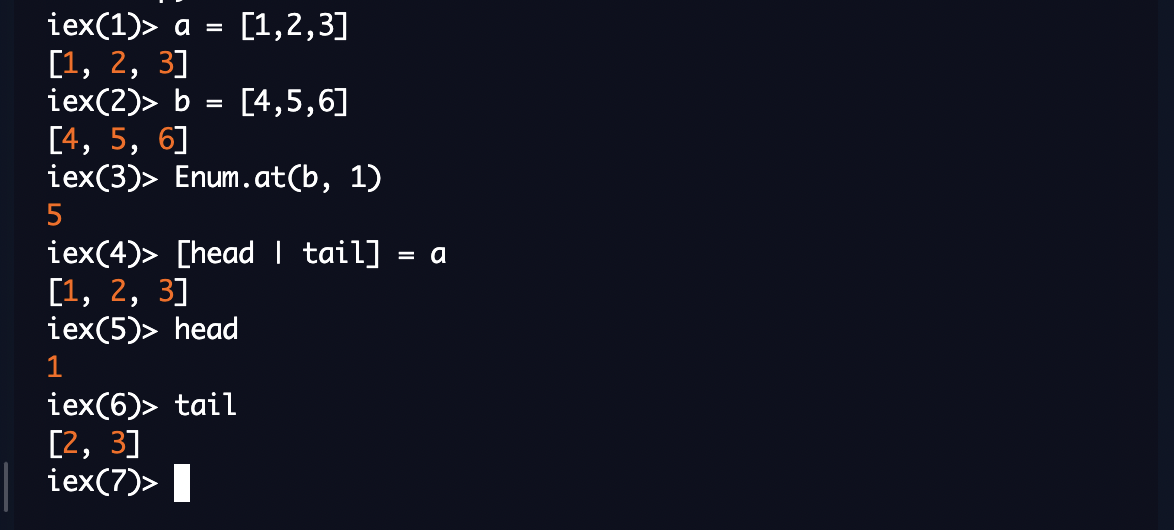Learn to Code via Tutorials on Repl.it!

← Back to all posts

# Functional Programming Tutorial: Pascal Triangle in Elixir

Writing code to generate the Pascal triangle is a common coding problem.
The Pascal triangle looks like this:

``````          1
1   1
1   2   1
1   3   3   1
1   4   6   4   1
...``````

Note: The code for this has been provided in the excellent book, Introducing Elixir by Simon St. Laurent and J. David Eisenberg. You can refer to their Github.

This tutorial is primarily to explain & document the process of developing the function for the Pascal triangle as a beginner in Elixir and Functional Programming.

## Creating the Module

Create a new elixir file 'pascal.ex'. Let's create our module and give it a description.

``````defmodule Pascal do
@moduledoc"""
Code for generating a Pascal triangle as a list of lists when the number of rows in the triangle is passed as an input to the function.

By: Sam
Date: July 6, 2021
"""

@vsn 0.1

end``````

## Writing the main function - pascal_triangle

We need to create a function that will accept a positive integer as input and return a list of lists as the output.
We use the `@spec` to specify that the 'pascal_triangle' function will accept an integer (int()) as the input and return a list (list()) as the output.

(Note: A list of lists, is simply a list.)

``````defmodule Pascal do
@moduledoc"""
Code for generating a Pascal triangle as a list of lists when the number of rows in the triangle is passed as an input to the function.

By: Sam
Date: July 6, 2021
"""

@vsn 0.1

@spec (integer()) :: list()
def pascal_triangle(num) do
#we will come back to this
end

end``````

### Guards and a catch-all clause for pascal_triangle

We've specified that the input should be an integer, however, it also needs to be >=1, we can't create a pascal triangle with -2 rows. Let's add a guard clause and a catch-all function clause for when the user enters an invalid input. Let's also add function documentation.

``````defmodule Pascal do
@moduledoc"""
Code for generating a Pascal triangle as a list of lists when the number of rows in the triangle is passed as an input to the function.

By: Sam
Date: July 6, 2021
"""

@vsn 0.1

@doc"""
The pascal_triangle function takes a positive integer as input and returns a list where each element is a list representing a row of the pascal triangle and the number of elements is equal to the input provided by the user.

## Examples

pascal_triangle(1) returns []
pascal_triangle(2) returns [, [1,1]]
pascal_triangle(3) returns [, [1,1], [1,2,1]]

Each element of the pascal triangle is sum of the corresponding element and previous element in the previous row. i.e. the 2nd element in the third row will be the sum of the 1st and 2nd elements in the second row and so on.
"""
@spec pascal_triangle(integer()) :: list()
def pascal_triangle(num) when num>=1 do
#we will come back to this
end

def pascal_triangle(_num) do
end

end``````

Let's check if we're able to view the module and function documentation. In your shell type `elixirc pascal.ex` to compile the Pascal module and then run `iex`.Let's call the `h` commands on the Pascal module and the pascal_triangle function to view our documentation.## Doing the work - the pascal_recursion function

We can get to writing the main function now!

In functional programing, we use recursive functions rather than 'for' loops to iterate over a range or items in a list.

Few things to note:

• Since we've already included a guard for number of rows >=1, our first element of the final list is known - .
• Now for every row number till the input, we need to add a list of elements so that the final result is a list containing n lists (where n was the input)

Essentially, we need to count up from 1 to the number of rows input by the user, while adding a list for each count. Let's create a function for this. The pascal_triangle should call this new function by giving it the beginning arguments - counter = 1, rows = user input, and list = []

(Since our output should be a list of lists, we nest the 1 here in another list - our first element of the ultimate output is ``, not `1`)

``````defmodule Pascal do
@moduledoc"""
Code for generating a Pascal triangle as a list of lists when the number of rows in the triangle is passed as an input to the function.

By: Sam
Date: July 6, 2021
"""

@vsn 0.1

@doc"""
The pascal_triangle function takes a positive integer as input and returns a list where each element is a list representing a row of the pascal triangle and the number of elements is equal to the input provided by the user.

## Examples

pascal_triangle(1) returns []
pascal_triangle(2) returns [, [1,1]]
pascal_triangle(3) returns [, [1,1], [1,2,1]]

Each element of the pascal triangle is sum of the corresponding element and previous element in the previous row. i.e. the 2nd element in the third row will be the sum of the 1st and 2nd elements in the second row and so on.
"""
@spec pascal_triangle(integer()) :: list()
def pascal_triangle(num) when num>=1 do
pascal_recursion(1, num, [])
end

def pascal_triangle(_num) do
end

def pascal_recursion(counter, num, list) when counter<= num do
#We will come back to this
end

def pascal_recursion(_counter, _num, list) do
list
end

end``````

As earlier, we have created two function clauses - we want the recursion to occur when the counter is less than or equal to the total number of rows and the output to be returned when the counter exceeds the total number of rows. Since, the recursion is not called again in this second clause, this breaks the recursive loop. Also, note the underscores ('_') before 'counter' and 'num' in the second pascal_recursion clause. Since, we're not using these variables to generate the output in this function clause, the interpreter will give an 'unused variable' warning if we don't add the underscores.

### Core of the pascal_recursion

Let's build our pascal_recursion function now. Few things to note:

• Each row is a list
• The number of elements in a row is equal to the row index i.e. the 1st row has 1 element (), the 2nd row has 2 elements ([1,1]), the 3rd row has 3 elements ([1,2,1]) and so on.
• The xth element of a row is the sum of the xth and (x-1)th element of the previous row.
• The 1st element of a row is the sum of 1st and 0th element of the previous row. The 0th element can be considered 0, so we get the first element as 1 + 0 = 1 for every row
• Similarly, the last element of a row, say 6th element of 6th row is sum of 6th element + 5th element of fifth row. But the fifth row does not have a 6th element, which can thus be considered as 0.
• List element positions in Elixir start with 0, similar to other programming languages like python
• To get the element at index n in a list, we can use `Enum.at(listname, n)` or `Enum.fetch(list, n)` or `Enum.fetch!(listname, n)` (Reference)
• To get the first element from a list, we can use pattern matching as follows `list 1 = [head | tail]`. the first element of list 1 will bind to `head` and the rest of the elements will bind (as a list of elements) to the `tail`

Let's try some of the code above for better understanding.
In your iex shell, create a list `a = [1,2,3]` and another `b = [4,5,6]`.

• to get the second element in b, we use `Enum.at(b, 1)`
• to get the head & tail of a, we can do `[head | tail] = a`For every recursion in the pascal_recursion function, we will:

• specify that the length of the new row should be equal to the counter value + 1
• get the reference list (list corresponding to previous row of the triangle from the list of lists)
• add the requisite number of elements to the empty list
• add the new list to the existing list of lists
• Call the recursion function again with the updated counter and list of lists.
``````defmodule Pascal do
@moduledoc"""
Code for generating a Pascal triangle as a list of lists when the number of rows in the triangle is passed as an input to the function.

By: Sam
Date: July 6, 2021
"""

@vsn 0.1

@doc"""
The pascal_triangle function takes a positive integer as input and returns a list where each element is a list representing a row of the pascal triangle and the number of elements is equal to the input provided by the user.

## Examples

pascal_triangle(1) returns []
pascal_triangle(2) returns [, [1,1]]
pascal_triangle(3) returns [, [1,1], [1,2,1]]

Each element of the pascal triangle is sum of the corresponding element and previous element in the previous row. i.e. the 2nd element in the third row will be the sum of the 1st and 2nd elements in the second row and so on.
"""
@spec pascal_triangle(integer()) :: list()
def pascal_triangle(num) when num>=1 do
pascal_recursion(1, num, [])
end

def pascal_triangle(_num) do
end

def pascal_recursion(counter, num, list) when counter< num do

counter = counter + 1
new_row_length = counter
[previous_row | _tail] = list

#new_row - we will come back to this

new_list = [new_row] ++ list
pascal_recursion(counter, num, new_list)
end

def pascal_recursion(_counter, _num, list) do
list
end

end``````

We still need to add code to create the actual list of elements corresponding to new_row. Notice that the pascal_triangle function in effect uses two nested recursions - once you iterate over the counter numbers till you reach the number of rows. Within each iteration, you iterate over the elements in the previous row to get the new row. In Python, you could implement this using nested for loops.

``````def pascal_triangle(num):
if type(num) == int and num >= 1:
main_list = []
counter = 1

for item in range(2, num+1):
#print(f'item is {item}')
new_row = []

for element in range(0, item):
#print(f'element is {element}')

if element>=1:
n1 = main_list[item-2][element-1]
else:
n1 = 0
#print(f'n1 for element {element} in the row {item} is {n1}')
if element<(item-1):
n2 = main_list[item-2][element]
else:
n2 = 0
#print(f'n2 for element {element} in the row {item} is {n2}')

#print(f'element {element} in the row {item} is {n1 + n2}')

new_row.append(n1 + n2)
#print(f' row number {item} is now {new_row}')

#print(f'row number {item} is finally {new_row}')

main_list.append(new_row)
counter += 1
#print(f' main_list is finally {main_list} ')

return main_list

new = input("Enter the number of rows in the pascal triangle: ")
print(pascal_triangle(int(new)))``````

Since we're using recursive functions instead of for loops in elixir, let's create our final function that generates each row.

## The row_creator function

Before we get to the code, let's try it out here:
For row 2,

• the number of elements = row number = 2
• the 1st element of row 2 = 0th element + 1st element of row 1 i.e. nth element of a row is nth element + (n-1)th element of previous row.

We pass the following arguments to the row_creator function:

• 'element' - which refers to the index of the element in the new row. Since list indexes start from 0, we have set this as 0.
• 'row_length' - the number of elements in the row, which is equal to the current value of the 'counter' in the pascal_recursion function
• 'previous_row' - the list of elements corresponding to the previous row of the pascal triangle, which will be used to build our new row
• 'new_row' - the list to which wwe keep adding elements. We start off with passing an empty list for this argument in the pascal_recursion function

The row_creator function also has two clauses - we want a new element to be created when the element index is less than or equal to the row_length-1. In the second clause, we simply return the list corresponding to new_row without making any changes.

``````defmodule Pascal do
@moduledoc"""
Code for generating a Pascal triangle as a list of lists when the number of rows in the triangle is passed as an input to the function.

By: Sam
Date: July 6, 2021
"""

@vsn 0.1

@doc"""
The pascal_triangle function takes a positive integer as input and returns a list where each element is a list representing a row of the pascal triangle and the number of elements is equal to the input provided by the user.

## Examples

pascal_triangle(1) returns []
pascal_triangle(2) returns [, [1,1]]
pascal_triangle(3) returns [, [1,1], [1,2,1]]

Each element of the pascal triangle is sum of the corresponding element and previous element in the previous row. i.e. the 2nd element in the third row will be the sum of the 1st and 2nd elements in the second row and so on.
"""
@spec pascal_triangle(integer()) :: list()
def pascal_triangle(num) when num>=1 do
pascal_recursion(1, num, [])
end

def pascal_triangle(_num) do
end

def pascal_recursion(counter, num, list) when counter< num do
#IO.puts("The current final list is")
#IO.inspect(list)
counter = counter + 1
new_row_length = counter
[previous_row | _tail] = list
#IO.puts("current counter is #{counter}")
#IO.puts("The previous row currently is")
#IO.inspect(previous_row)
new_row = row_creator(0, new_row_length, previous_row, [])
#IO.puts("the new row currently is")
#IO.inspect(new_row)
new_list = [new_row] ++ list
pascal_recursion(counter, num, new_list)
end

def pascal_recursion(_counter, _num, list) do
list |> Enum.reverse()
end

def row_creator(element, row_length, previous_row, new_row) when element<=row_length-1 do
n1 = cond do
element<1 -> 0
element>=1 -> Enum.at(previous_row, element-1)
end
#IO.puts("n1 is #{n1}")

n2 = cond do
element<=row_length-2 -> Enum.at(previous_row, element)
element>row_length-2 -> 0
end

#IO.puts("n2 is #{n2}")
new_elem = n1 + n2
updated_row = new_row ++ [new_elem]
row_creator(element+1, row_length, previous_row, updated_row)

end

def row_creator(_element, _row_length, _previous_row, new_row) do
new_row
end

end``````

You can see code commented out in various line - I used this to debug and arrive at the final result.

Finally, notice that in the second clause of pascal_recursion (which is implemented when the counter = number of rows), we pass the final list to `Enum.reverse()`. This is because we have been adding new rows to the left of previous rows when we do `new_list = [new_row] ++ list` in the pascal_recursion function. To reverse the order of the nested lists, we use `Enum.reverse()`

Compile the file again by typing `elixirc pascal.ex` in the shell. Let's check if our function works. We'll add some code to main.exs so that the function is called when we run the repl.

``````rows = IO.getn("Please enter the number of rows for the pascal triangle: ")

String.to_integer(rows) |> Pascal.pascal_triangle() |> IO.inspect()
# You can also write the above line as follows:
#IO.inspect(Pascal.pascal_triangle(String.to_integer(rows)))``````

Elixir allows you to pass arguments from one function to another in a linear manner, rather than using nested functions or having to create intermediate variables.

Let's run our repl!Phew! This was one long tutorial. Hit me up with comments or any suggestions on improving the code.